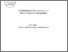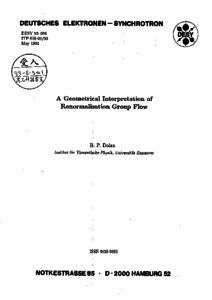# A Geometrical Interpretation Of Renormalisation Group Flow

Dolan, Brian P. (1994) A Geometrical Interpretation Of Renormalisation Group Flow. International Journal of Modern Physics A, 09 (08). pp. 1261-1286. ISSN 0217-751XPreviewmore...Add this article to your Mendeley library

## Abstract

The renormalization group (RG) equation in D-dimensional Euclidean space, RD, is analyzed from a geometrical point of view. A general form of the RG equation is derived which is applicable to composite operators as well as tensor operators (on RD) which may depend on the Euclidean metric. It is argued that physical N-point amplitudes should be interpreted as rank N covariant tensors on the space of couplings, , and that the RG equation can be viewed as an equation for Lie transport on with respect to the vector field generated by the β functions of the theory. In one sense it is nothing more than the definition of a Lie derivative. The source of the anomalous dimensions can be interpreted as being due to the change of the basis vectors on under Lie transport. The RG equation acts as a bridge between Euclidean space and coupling constant space in that the effect on amplitudes of a diffeomorphism of RD (that of dilations) is completely equivalent to a diffeomorphism of generated by the β functions of the theory. A form of the RG equation for operators is also given. These ideas are developed in detail for the example of massive λφ4 theory in four dimensions.

Item Type: Article Energy-Momentum Tensor; Scalar Field-Theory; C-Theorem; Faculty of Science and Engineering > Theoretical Physics 12509 https://doi.org/10.1142/S0217751X94000571 Dr. Brian Dolan 03 Mar 2020 17:08 International Journal of Modern Physics A World Scientific YesItem control page